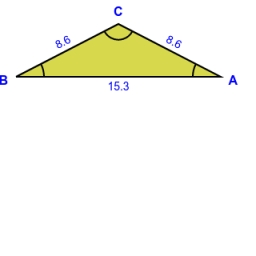# Isosceles triangle

Calculate the height of the isosceles triangle ABC with the base AB, AB = c = 10 cm and the arms a = b = 13 cm long.

v =  12 cm

### Step-by-step explanation:

Try calculation via our triangle calculator.Did you find an error or inaccuracy? Feel free to write us. Thank you!

Tips for related online calculators
The Pythagorean theorem is the base for the right triangle calculator.